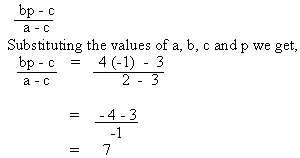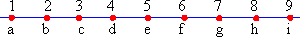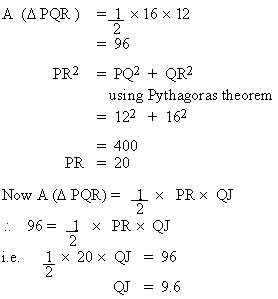Support the Monkey! Tell All your Friends and TeachersHome MonkeyNotes Printable Notes Digital Library Study Guides Study Smart Parents Tips College Planning Test Prep Fun Zone Help / FAQ How to Cite New Title Request

 Let the two numbers be a and b. a + b = 2 (a - b) a + b = 2a - 2b           3b = a but       b= 10            a = 30 Ans : E The given expression isAns : C n (A È B) = n(A) + n(B) - n(A Ç B) But u = n (A È B) + n (A È B) n ( A È B) = 42 + 45 - 22 n (A È B) = 65 85 = 65 + n (A È B) n (A È B) = 20 Ans : BSince the letters represent consecutive integers, moving backwards from f to b one gets 4 units. \ f - b = 6 - 2 = 4 Ans : BSince the letters represent consecutive integers, the sum c + d + g = 3c + 5 \ c + d + g = c + c + 1 + c + 4 = 3c + 5 Ans : ASince the letters represent consecutive integers, consider b = a + 1, c = a + 2, and d = c + 1 To find d2 - b2 Substituting the values of d as (c + 1) and b as (a + 1) (c + 1)2 - (a + 1)2 = c2 + 2c + 1 - a2 - 2a - 1 = c2 - a2 + 2 ( c - a) = 24 + 2 ( c - a) [Since c2 - a2 = 24] But c = a + 2 \ d2 - b2 = 28 Ans : EAns : C Index Test 3 Section 1: Verbal Reasoning Section 2: Mathematical Reasoning Section 3: Verbal Reasoning Section 4: Mathematical Reasoning Section 5: Reading Comprehension Section 6: Mathematical Reasoning Section 7: Mathematical Reasoning Answer Key To Test 3 Answer Explanation To Test 3 Section 1: Verbal Reasoning Section 2: Mathematical Reasoning Section 3: Verbal Reasoning Section 4: Mathematical Reasoning Section 5: Reading Comprehension Section 6: Mathematical Reasoning Section 7: Mathematical Reasoning Test 4
 Search: All Products Books Popular Music Classical Music Video DVD Toys & Games Electronics Software Tools & Hardware Outdoor Living Kitchen & Housewares Camera & Photo Cell Phones Keywords: